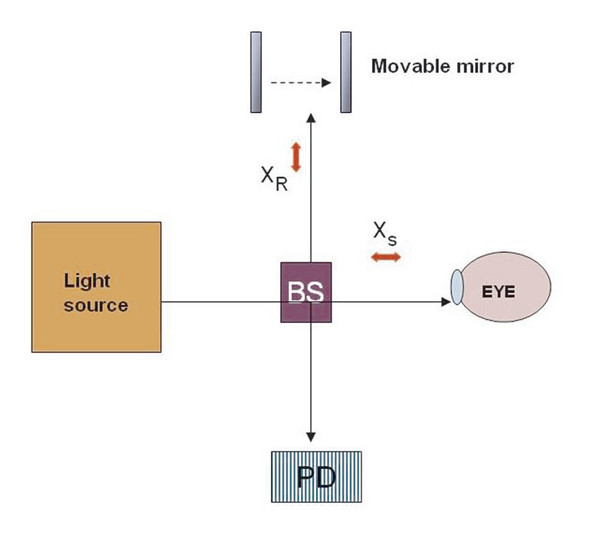# 2 Time-Domain and Fourier-Domain Optical Coherence Tomography

10.1055/b-0035-121717

## 2 Time-Domain and Fourier-Domain Optical Coherence Tomography

Optical coherence tomography (OCT) has revolutionized the investigative field of ophthalmology in the last two decades. Huang et al established OCT evaluation of the retina using infrared light wavelength in 1991. 1 Time-domain (TD) OCT was the first model of in vivo evaluation of the human retina and optic nerve until 1995, when Fourier- or frequency-domain (FD) OCT was introduced for ocular imaging. 2 The mechanisms by which the TD and FD OCT functions have been studied widely since then and have been used accordingly for various clinical conditions. The popularity of the method lies in its ability to delineate micrometer differences or variations in the clinical tissues like macula or cornea. Although TD and FD OCT were introduced in the early 1990s, FD OCT has been applied widely only after 2000. Wojtkowski et al were among the earliest to report an experimental study showing the superior sensitivity of FD over TD OCT. 2

This chapter presents the basic optical differences between the TD and FD OCT systems. We also mention various studies that have demonstrated the sensitivity differences between the methods. 3 ,​ 4 ,​ 5 ,​ 6 The advantages and limitations of the individual methods are also addressed. appropriately.

### 2.1 Optics: How They Differ

In a conventional TD system, the source light passes through the beam splitter, which splits the light beam into sample and reference. The sample arm is directed to the sample or tissue end and the other to the moving reference mirror (Fig. 2.1). The light beams, which are backscattered and back-reflected from the sample and the reference mirror are rechanneled by the beam splitter and detected by the photodetector. Interference can occur only when the two arms are matched in length so that the returning pulses can arrive at the detector at the same time to interfere. Yaqoob et al reported the bio-optical differences between the two domains of OCTs and have shown the theoretical differences. 3Fig. 2.1 Schematic diagram showing the optical principle of time-domain optical coherence tomography. BS, beam splitter; PD, photodetector; XR, reference arm interface signal; XS, sample arm interface signal.

#### 2.1.1 Time-Domain OCT

In a TD OCT system, the reference arm is typically displaced and scanned over a distance equal to the depth range, x depth that is to be probed for the sample. 3 For a given total scan time duration, T, we can determine that a TD OCT system will spend a duration (L C/ x depth) × T of collecting interference signal from any given interface within the sample, where L C is the coherence length. 3 A determination of the TD OCT sensitivity can be made by calculating the number of useful signal photons within that duration and comparing that with the noise photon count. The total useful interference signal photons that will be collected in this context are given by the following equation:

SignalTDOCT=2√PrefPsigLC*T/xdepthε/hv{Signal}_{TDOCT}=2\surd \left({P}_{ref}{P}_{sig}\right)\left({L}_{{C}^{{*}}}T/{x}_{depth}\right)\left(\epsilon /hv\right)

where P ref and P sig are the collected reference and sample power at wavelength k, respectively, and ε is the quantum efficiency of the detector and hv is the photon quantum energy. 3 The noise count is given by the square root of the total photons that are detected by the detector during that time duration. As the reference power P ref typically dominates over all other signal in TD OCT, noise is calculated as follows:

NoiseTDOCT=√PrefLC* T/xdepth ε/hv{Noise}_{TDOCT}=\surd \left\{{P}_{ref}\left({L}_{{C}^{{*}}}{ }T/{x}_{depth}\right){ }\left(\epsilon /hv\right)\right\}

Although the photodetector is collecting backscattered photons from the interface of interest for the entire duration of the acquisition, T, the detection scheme is actively selecting only those photons for OCT signal construction over a much shorter duration, L C* T/ x depth. This necessarily leads to diminished signal sensitivity compared with a scheme that is capable of using those photons for OCT signal construction over the entire duration of the acquisition (example FD OCT).

#### 2.1.2 Fourier-Domain OCT

In an FD system, the source light passes through the beam splitter, which splits the light beam into sample and reference. The sample arm is directed to the sample or tissue end and the other to the stationary reference mirror (Fig. 2.2). The light beams that are backscattered and back-reflected from the sample and the reference mirror are rechanneled by the beam splitter and detected by the spectrometer. There is an immobile reference mirror, and instead of a photodetector as in TD OCT, there is a spectrometer (Fig. 2.2). In FD OCT, the spectral variation in the detected signal is analyzed by the low-loss spectrometer. An interface that gives a smaller value (X RX S) will produce a slower sinusoidal spectral oscillation than will an interface with a larger value (X RX S). Therefore, a Fourier transform of the spectral measurement will produce a line-scan profile similar to that obtained from TD OCT. It should be noted that in FD OCT, signal contribution from two or more interfaces may be collected simultaneously as they contribute to different spectral oscillation components. 3 Here the total useful interference signal photons from a given interface collected in this context are given by the following equation:

SignalFDOCT=2√Pref Psig T*ε/hv{Signal}_{FDOCT}=2\surd \left(\left({P}_{ref}{ }{P}_{sig}\right){ }\left(T{*}\epsilon /hv\right)\right)Get Clinical Tree app for offline access